xMagnetic quantum numberEncyclopedia
In atomic physics
Atomic physics
Atomic physics is the field of physics that studies atoms as an isolated system of electrons and an atomic nucleus. It is primarily concerned with the arrangement of electrons around the nucleus and...

, the magnetic quantum number is the third of a set of quantum number
Quantum number
Quantum numbers describe values of conserved quantities in the dynamics of the quantum system. Perhaps the most peculiar aspect of quantum mechanics is the quantization of observable quantities. This is distinguished from classical mechanics where the values can range continuously...

s (the principal quantum number
Principal quantum number
In atomic physics, the principal quantum symbolized as n is the firstof a set of quantum numbers of an atomic orbital. The principal quantum number can only have positive integer values...

, the azimuthal quantum number
Azimuthal quantum number
The azimuthal quantum number is a quantum number for an atomic orbital that determines its orbital angular momentum and describes the shape of the orbital...

, the magnetic quantum number, and the spin quantum number
Spin quantum number
In atomic physics, the spin quantum number is a quantum number that parameterizes the intrinsic angular momentum of a given particle...

) which describe the unique quantum state of an electron and is designated by the letter m. The magnetic quantum number denotes the energy levels available within a subshell.

## Derivation

There are a set of quantum numbers associated with the energy states of the atom. The four quantum numbers n, , m, and s specify the complete and unique quantum state of a single electron in an atom called its wavefunction
Wavefunction
Not to be confused with the related concept of the Wave equationA wave function or wavefunction is a probability amplitude in quantum mechanics describing the quantum state of a particle and how it behaves. Typically, its values are complex numbers and, for a single particle, it is a function of...

or orbital
Atomic orbital
An atomic orbital is a mathematical function that describes the wave-like behavior of either one electron or a pair of electrons in an atom. This function can be used to calculate the probability of finding any electron of an atom in any specific region around the atom's nucleus...

. The wavefunction of the Schrödinger wave equation
Schrödinger equation
The Schrödinger equation was formulated in 1926 by Austrian physicist Erwin Schrödinger. Used in physics , it is an equation that describes how the quantum state of a physical system changes in time....

reduces to the three equations that when solved lead to the first three quantum numbers. Therefore, the equations for the first three quantum numbers are all interrelated. The magnetic quantum number arose in the solution of the azimuthal part of the wave equation as shown below.

The magnetic quantum number associated with the quantum state is designated as m. The quantum number m refers, loosely, to the direction of the angular momentum
Angular momentum
In physics, angular momentum, moment of momentum, or rotational momentum is a conserved vector quantity that can be used to describe the overall state of a physical system...

vector. The magnetic quantum number m does not affect the electron's energy, but it does affect the probability cloud. Given a particular , m is entitled to be any integer from - up to . More precisely, for a given orbital momentum quantum number (representing the azimuthal quantum number
Azimuthal quantum number
The azimuthal quantum number is a quantum number for an atomic orbital that determines its orbital angular momentum and describes the shape of the orbital...

associated with angular momentum), there are 2+1 integral magnetic quantum numbers m ranging from - to , which restrict the fraction of the total angular momentum along the quantization axis so that they are limited to the values m. This phenomenon is known as space quantization. It was first demonstrated by two German physicists, Otto Stern
Otto Stern
Otto Stern was a German physicist and Nobel laureate in physics.-Biography:Stern was born in Sohrau, now Żory in the German Empire's Kingdom of Prussia and studied at Breslau, now Wrocław in Lower Silesia....

and Walther Gerlach.
Since each electronic orbit has a magnetic moment in a magnetic field the electronic orbit will be subject to a torque which tends to make the vector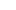parallel to the field. The precession of the electronic orbit in a magnetic field is called the Larmor precession
Larmor precession
In physics, Larmor precession is the precession of the magnetic moments of electrons, atomic nuclei, and atoms about an external magnetic field...

.

To describe the magnetic quantum number m you begin with an atomic electron's angular momentum, L, which is related to its quantum number by the following equation: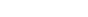where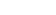is the reduced Planck constant. The energy of any wave is the frequency multiplied by Planck's constant. This causes the wave to display particle-like packets of energy called quanta
Quantum
In physics, a quantum is the minimum amount of any physical entity involved in an interaction. Behind this, one finds the fundamental notion that a physical property may be "quantized," referred to as "the hypothesis of quantization". This means that the magnitude can take on only certain discrete...

. To show each of the quantum numbers in the quantum state, the formulae for each quantum number include Planck's reduced constant which only allows particular or discrete or quantized energy levels.

To show that only certain discrete amounts of angular momentum are allowed, has to be an integer. The quantum number m refers to the projection of the angular momentum for any given direction, conventionally called the z direction. Lz, the component of angular momentum in the z direction, is given by the formula: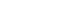Another way of stating the formula for the magnetic quantum number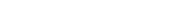is the eigenvalue, Jz=mh/2π.

Where the quantum number is the subshell, the magnetic number m represents the number of possible values for available energy levels of that subshell as shown in the table below.
Relationship between Quantum Numbers
Orbital Values Number of Values for m
s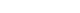1
p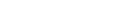3
d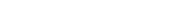5
f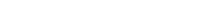7
g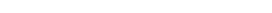9

The magnetic quantum number determines the energy shift of an atomic orbital
Atomic orbital
An atomic orbital is a mathematical function that describes the wave-like behavior of either one electron or a pair of electrons in an atom. This function can be used to calculate the probability of finding any electron of an atom in any specific region around the atom's nucleus...

due to an external magnetic field, hence the name magnetic quantum number (Zeeman effect
Zeeman effect
The Zeeman effect is the splitting of a spectral line into several components in the presence of a static magnetic field. It is analogous to the Stark effect, the splitting of a spectral line into several components in the presence of an electric field...

).

However, the actual magnetic dipole moment of an electron in an atomic orbital arrives not only from the electron angular momentum, but also from the electron spin, expressed in the spin quantum number
Spin quantum number
In atomic physics, the spin quantum number is a quantum number that parameterizes the intrinsic angular momentum of a given particle...

.

• Quantum number
Quantum number
Quantum numbers describe values of conserved quantities in the dynamics of the quantum system. Perhaps the most peculiar aspect of quantum mechanics is the quantization of observable quantities. This is distinguished from classical mechanics where the values can range continuously...

• Azimuthal quantum number
Azimuthal quantum number
The azimuthal quantum number is a quantum number for an atomic orbital that determines its orbital angular momentum and describes the shape of the orbital...

• Principal quantum number
Principal quantum number
In atomic physics, the principal quantum symbolized as n is the firstof a set of quantum numbers of an atomic orbital. The principal quantum number can only have positive integer values...

• Spin quantum number
Spin quantum number
In atomic physics, the spin quantum number is a quantum number that parameterizes the intrinsic angular momentum of a given particle...

• Total angular momentum quantum number
• Basic quantum mechanics
• Bohr atom
• Schrödinger equation
Schrödinger equation
The Schrödinger equation was formulated in 1926 by Austrian physicist Erwin Schrödinger. Used in physics , it is an equation that describes how the quantum state of a physical system changes in time....